# High School Math : Kites

## Example Questions

### Example Question #1 : How To Find The Area Of A Kite

What is the area of a kite with diagonals of 5 and 7?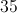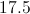Explanation:

To find the area of a kite using diagonals you use the following equation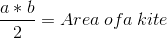That diagonals (and)are the lines created by connecting the two sides opposite of each other.

Plug in the diagonals forandto get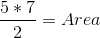Then multiply and divide to get the area.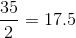The answer is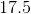### Example Question #2 : How To Find The Area Of A Kite

Find the area of the following kite: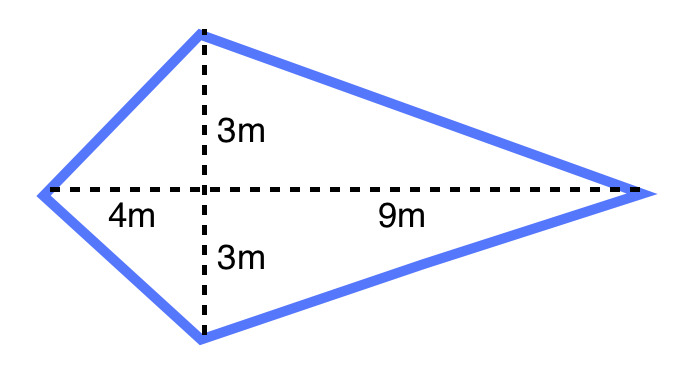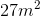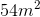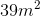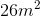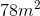Explanation:

The formula for the area of a kite is: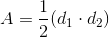Where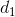is the length of one diagonal and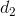is the length of the other diagonal

Plugging in our values, we get: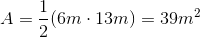### Example Question #3 : How To Find The Area Of A Kite

Find the area of the following kite: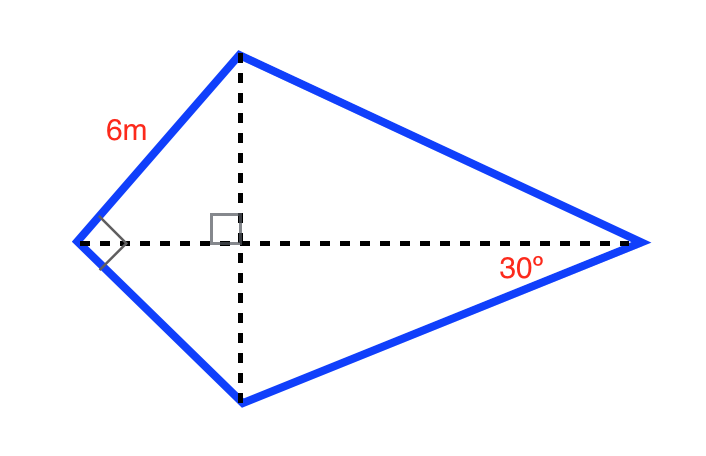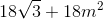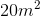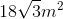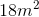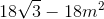Explanation:

The formula for the area of a kite is: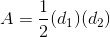whereis the length of one diagonal andis the length of another diagonal.

Use the formulas for a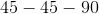triangle and a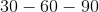triangle to find the lengths of the diagonals. The formula for atriangle is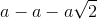and the formula for atriangle is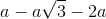.

Ourtriangle is: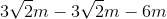Ourtriangle is: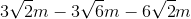Plugging in our values, we get: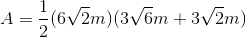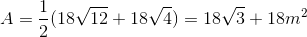### Example Question #1 : Quadrilaterals

Find the perimeter of the following kite: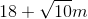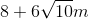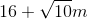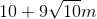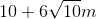Explanation:

In order to find the length of the two shorter edges, use a Pythagorean triple: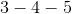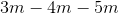In order to find the length of the two longer edges, use the Pythagorean theorem: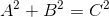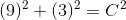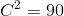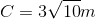The formula of the perimeter of a kite is: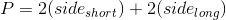Plugging in our values, we get: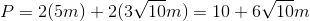### Example Question #2 : Quadrilaterals

Find the perimeter of the following kite: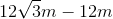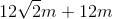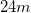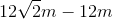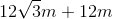Explanation:

The formula for the perimeter of a kite is: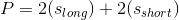Where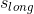is the length of the longer side and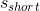is the length of the shorter side

Use the formulas for atriangle and atriangle to find the lengths of the longer sides. The formula for atriangle isand the formula for atriangle is.

Ourtriangle is:Ourtriangle is:Plugging in our values, we get: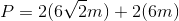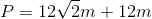### All High School Math Resources